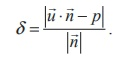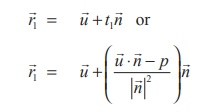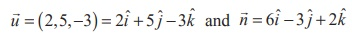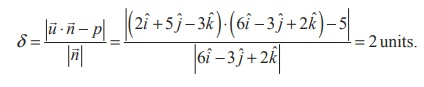Home | | Maths 12th Std | Distance of a point from a plane

# Distance of a point from a plane

Distance of a point from a plane (a) Vector form of equation (b) Cartesian form of equation

Distance of a point from a plane

## (a) Vector form of equation

### Theorem 6.20

The perpendicular distance from a point with position vectorto the plane= p is given by### Proof

Let A be the point whose position vector is.

Let F be the foot of the perpendicular from the point A to the plane. The line joining F and A is parallel to the normal vectorand hence its equation is+ t.

But F is the point of intersection of the line=+ tand the given planep . If1 is the position vector of F, thenfor some t1R, andEliminating1 we getTherefore, the length of the perpendicular from the point A to the given plane isThe position vector of the foot F of the perpendicular AF is given by## (b) Cartesian form of equation

In Caretesian form if A( x1 , y1 , z1 ) is the given point with position vectorand ax + by + cz = p is the Cartesian equation of the given plane, then= x1ˆi  + y1ˆ j + z1ˆk and  n = aˆi  + bˆj + cˆk.  Therefore, using these vectors inwe get the perpendicular distance from a point to the plane in Cartesian form as### Remark

The perpendicular distance from the origin to the plane ax + by + cz + d = 0 is given by### Example 6.49

Find the distance of a point (2, 5, −3) from the plane(6ˆi − 3ˆj + 2ˆk ) = 5 .

### Solution

Comparing the given equation of the plane withp we have= − 3ˆ+ 2ˆ

We know that the perpendicular distance from the given point with position vectorto the planerp is given by.Therefore, substitutingin the formula, we get### Example 6.50

Find the distance of the point (5, −5, −10) from the point of intersection of a straight line passing through the points A(4,1, 2) and B (7, 5, 4) with the plane x y + z = 5 .

### Solution

The Cartesian equation of the straight line joining A and B isTherefore, an arbitrary point on the straight line is of the form (3t + 4, 4t +1, 2t + 2) . To find the point of intersection of the straight line and the plane, we substitute x = 3t + 4, y = 4t +1, z = 2t + 2  in x y + z = 5 , and we get t = 0 . Therefore,the point of intersection of the straight line is (2, −1, 2) .

Now, the distance between the two points (2, −1, 2) and (5, −5, −10) isTags : Definition, Theorem, Proof, Solved Example Problems, Solution , 12th Mathematics : UNIT 6 : Applications of Vector Algebra
Study Material, Lecturing Notes, Assignment, Reference, Wiki description explanation, brief detail
12th Mathematics : UNIT 6 : Applications of Vector Algebra : Distance of a point from a plane | Definition, Theorem, Proof, Solved Example Problems, Solution